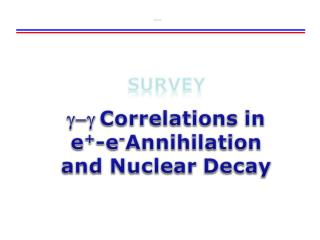DownloadDownload PresentationSizes

Sizes

Télécharger la présentationSizes

- - - - - - - - - - - - - - - - - - - - - - - - - - - E N D - - - - - - - - - - - - - - - - - - - - - - - - - - -
Presentation Transcript

1. Sizes

2. dN/dEg dN/dEg Eg/2E0 1 Eg/2E0 1 Positronium and e+-e- Annihilation Para Positronium Ortho Positronium Decay at rest: 2Eg≈E0 ≈ 1.022 MeV2-body decay  q12≈1800 • q12 • 2-body decayline spectrumin Eg and q12 • 3-body decaycontinuumin Eg and q12 Gamma-Gamma Correlations

3. Radiation Detectors for Medical Imaging Positron emission tomographic (PET) virtual slice through patient’s brain Administer to patient radioactive water: H217O radioactive acetate: 11CH3COOX Observe 17O or 11C b+ decay by Positron e+ (anti-matter) annihilates with electron e- (its matter equivalent of the same mass) to produce pure energy (photons, g-rays). Energy and momentum balance require back-to-back (1800) emission of 2 g-rays of equal energy Gamma-Gamma Correlations g Detectors (NaI(Tl))

4. ANSEL Angular Correlation Experiment Gamma-Gamma Correlations Top left: PET imaging experiment setup with two 1.5”x1.5” NaI(Tl) detectors (BICRON) on a slotted correlation table.A “point-like” 22Na g source can be hidden from view.Top right: 22Na g spectrum measured with NaI1. Bottom right: g - gangular correlation measurement. Second correlation setup: NaI(Tl) vs. HPGe

5. E1-E2-Dt Multi-Parameter Measurement g-gcascade (60Ni) or PET experiment, full 3D energy-time distribution. PreAmp Amp Energy 1 Counter Counter Gate Generator TFA/CFTD DAQ Start Stop Relative Time TACDt Gate Generator Delay var. TFA/CFTD Gamma-Gamma Correlations Energy 2 PreAmp Amp Pulse Generator Pulser Counter Dead time measurement

6. E1-E2 Dual-Parameter Measurement g-gcascade (60Ni) or PET experiment, full 2D spectrum PreAmp Amp Energy 1 Gate Generator TFA/CFTD DAQ Strobe OR Coincid. Gate Generator Delay var. TFA/CFTD Gamma-Gamma Correlations Energy 2 PreAmp Amp Pulse Generator Pulser Counter Dead time measurement

7. 2D Parameter Measurement E1 2D Scatter Plot: Each point represents one event {E1, E2} Projection on E1 axis Gamma-Gamma Correlations E2 Projection on E2axis

8. Gated E1-E2 Coincidence Measurement g-gcascade (60Ni) or PET experiment, gates on g1 and g2 lines Energy 1 Amp Gate Generator SCA DAQ Strobe OR Coincid. Gate Generator Delay var. SCA Gamma-Gamma Correlations Energy 2 Amp Pulse Generator Pulser Counter Dead time measurement

9. Pre Amp Counter 1 Gate Generator 1 TFA/CFTD Counter Coinc. Coincidence Gate Generator TFA/CFTD 2 Counter 2 Pre Amp Absolute Activity Measurement Activity A [disintegrations/time], independent radiation types i =1,2 detection probabilities Pi N1 Activity A N12 N2 Gamma-Gamma Correlations

10. Sizes survey g-g Correlations in Nuclear Decay

11. Y (r) 0 r Bound States r Potential Symmetries of the Nuclear Mean Field Nucleon-nucleon forces are dominantly radial Gamma-Gamma Correlations n=2 n=1 r n=0

12. r Stationary Nuclear States Consequences of space-inversion and rotational invariance:Stationary states eigen states are also eigen states of Radial (r) and angular (q, f) degrees of freedom are independent  Separated.o.f in Schrödinger Equ. Product wave functions En Ip2+ 1- 0+ Spherical Harmonics or (axial symmetry)Legendre Polynomials Gamma-Gamma Correlations n=2 n=1 r n=0

13. Electromagnetic Nuclear Transitions Conserved: Total energy (E), total angular momentum (I) and total parity (p): Ip2+ Ip2+ Ei 1- 1- Ef 0+ 0+ Gamma-Gamma Correlations Consider here only electric multipole transitions.Neglect weaker magnetic transitions due to changes in current distributions.

14. t-Dependent Electromagnetic Radiation Fields Protons in nuclei = moving charges  emits elm. radiation Propagating ElectricDipole Field Heinrich Hertz Monopole ℓ= 0 Dipole ℓ = 1 Gamma-Gamma Correlations Quadrupole ℓ =2 Point Charges NuclearCharge Distribution E. Segré: Nuclei and Particles, Benjamin&Cummins, 2nd ed. 1977

15. z mi mf Selection Rules for Electromagnetic Transitions Conserved: Total energy (E), total angular momentum (I) and total parity (p): Quantization axis : z direction.Physical alignment of I possible (B field, angular correlation) Coupling of Angular Momenta mg Gamma-Gamma Correlations

16. z y q f x Spherical Harmonics P1|m|cos (mf) Plot Re YlmP1|m|cos (mf) Emission in z directionEmission perpend. to z Gamma-Gamma Correlations

17. g-g Angular Correlations Simple example: g cascade 0  1  0 Mostly Dm=±1 emitted in z-direction Det 1: Define z, select transition with maximum g intensity for q1=0. Det 2: Measure emission patterns with respect to this z direction  determine Dm. g1 Q1=0 Q2 Det 1 g2 Det 2 Gamma-Gamma Correlations General Expression for g-g angular correlation Typically: n ≤ 2. Legendre Polynomials Pn(cosq)

18. E2 g-g Angular Correlations Example: Rotational E2-g cascade, Dm=±2 maximally emitted in z-direction Anisotropy Gamma-Gamma Correlations After: G. Musiol, J. Ranft, R. Reif, D. Seeliger, Kern-u. Elementarteilchenphysik, VCH 1988

19. No More Correlations Gamma-Gamma Correlations

20. Gamma-Gamma Correlations